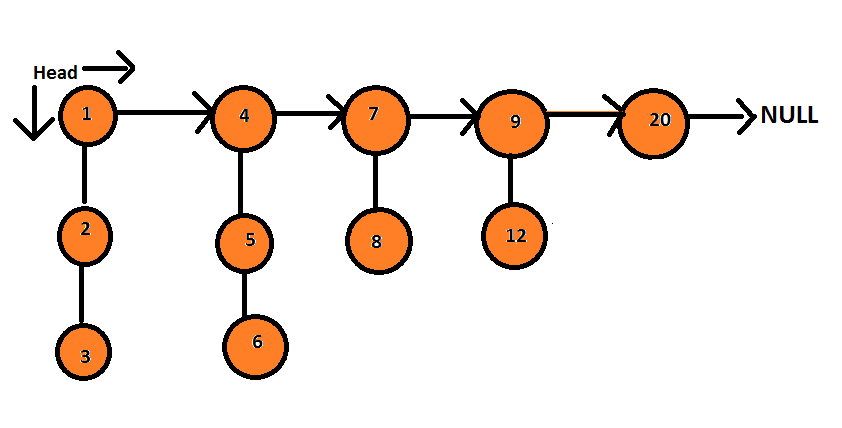Posted: 29 Jan, 2021
Difficulty: Easy

## PROBLEM STATEMENT

#### Your task is to flatten this linked such that all nodes appear in a single layer or level while the nodes should maintain the sorted order.

##### For example:
``````The given linked list is -
````````````So your output should be

1 → 2 → 3 → 4 → 5 → 6 → 7 → 8 → 9 → 12 → 20 → null.
``````
##### Note:
``````The flattened list will be printed using the bottom pointer instead of the next pointer.

The value of any node in the linked list will not be equal to -1.
``````
##### Input format :
``````The first line of input contains an integer ‘T’ denoting the number of test cases.

The first line of the test case contains ‘N’ which represents the number of next-nodes in the linked list.

The description of the next N lines is as follows-

Each line contains space-separated integers which are the child nodes of the head linked list and each line ends with -1 to indicate that the sublist is over. Thus, -1 will never be a linked list element.
``````
##### Output format :
``````For each test case, return the head node of the final linked list. The output of each test case will be printed in a separate line.
``````
##### Note:
``````You don’t have to print anything; it has already been taken care of. Just implement the given function.
``````
##### Constraints:
``````1 <= T <= 5
1 <= N <= 100
1 <= C <= 20
1 <= data <= 1000

Time Limit: 1sec
``````Approach 1

The idea is to use an extra array to first store all the elements of the linked list and then sort the array and finally put those elements back into the linked list and return.

• Declare an array ‘Answer’ to store the elements of the linked list.
• Run a while loop till the next of the linked list reaches NULL.